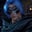Related Tags

golang
communitycreator

# How to iterate through a slice in GolangGutha Vamsi Krishna

In this shot, we will learn how to iterate through a slice in Golang.

A slice is a dynamically-sized array. It can grow or shrink if we add or delete items from it.

We can iterate through a slice using the for-range loop.

### Syntax

	for index, element := range slice {
//do something here
}


The range returns two values, which are the index and element of the current iteration.

If we don’t need to use an index, then we can use _, as shown below:

	for _, element := range slice {
//do something here
}


### Example

In this example, we will calculate the sum of the numbers in a slice by iterating through it.

### Code

package main

//program execution starts here
func main(){

//declare a slice of numbers
n := []int{10,20,30,40,50}

//declare a variable sum
sum := 0

//traverse through the slice
for _,element := range n{

sum += element
}

//display the sum
print(sum)
}
Demonstration of calculating the sum of the numbers in a slice

### Code explanation

• Line 4: Program execution in Golang starts from the main() function.

• Line 7: We declare and initialize the slice of numbers, n.

• Line 10: We declare and initialize the variable sum with the 0 value.

• Line 13: We traverse through the slice using the for-range loop. Since we are not using the index, we place a _ in that.

• Line 16: We add the present element to the sum.

• Line 20: We display the sum of the numbers in the slice.

RELATED TAGS

golang
communitycreator

CONTRIBUTORGutha Vamsi Krishna
RELATED COURSES

View all Courses

Keep Exploring

Learn in-demand tech skills in half the time